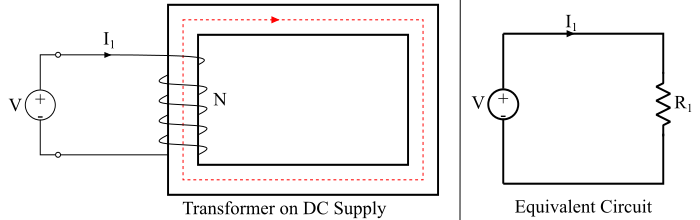# Why Don't Transformers Work on DC Supply?

Digital ElectronicsElectronElectronics & Electrical

Transformers do not work on DC supply. Let us see why.

The transformer works on the principle of mutual inductance i.e. current in one winding must change uniformly to induce the EMF in the other. When a DC voltage V is applied to the primary of a transformer, the primary will draw a constant current or direct current and hence, the magnetic flux produced in the core is also constant. As a result of it, there is not mutual inductance between the primary and secondary windings so no EMF will be induced in the primary and secondary windings because,

$$\mathrm{EMF,\:𝑒 = 𝑁\frac{𝑑_{φ}}{𝑑𝑡}}$$

Hence, the EMF is induced in the windings of the transformer only when a changing magnetic field links with the windings. Therefore, the transformer does not operate on DC supply.Also, connecting DC supply to the primary winding of the transformer can be dangerous. Refer the circuit diagram given in the figure, where, a DC voltage V is applied to the primary of the transformer so the DC primary current I1 flows in the primary circuit.

As the applied voltage is DC, so no self-induced EMF is produced in the primary winding to oppose the applied voltage V. The primary current is,

$$\mathrm{𝐼_{1} =\frac{𝑉}{𝑅_{1}}}$$

Where, R1 is the resistance of the primary winding.

Practically, the value of resistance (R1) of the primary winding is very small. Also, for DC supply, the inductive reactance XL is zero since the DC supply has no frequency. Hence, the total impedance of the primary winding is very small for DC supply.

Therefore, the primary winding will draw a very large current if DC supply is given to it. The result is that the primary winding will overheat and burn out or the fuses will blow.

Therefore, care must be taken not to connect the primary winding of the transformer across the DC supply.